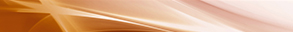# About this Journal

## Aims and scope

Applied Computational Intelligence and Soft Computing will focus on the disciplines of computer science, engineering, and mathematics. The scope of the journal includes developing applications related to all aspects of natural and social sciences by employing the technologies of computational intelligence and soft computing. The new applications of using computational intelligence and soft computing are still in development. Although computational intelligence and soft computing are established fields, the new applications of using computational intelligence and soft computing can be regarded as an emerging field, which is the focus of this journal.

The application areas of interest include but are not limited to:

• Interval Analysis (Real Interval Arithmetics, Complex Interval Arithmetics, Interval Equations, etc.)
• Interval Mathematics (Metric Topology for Intervals, Interval Integrals, Interval Differential Equations, etc.)
• Interval Computation (Matrix Computation with Intervals, Systems of Interval Equations, etc.)
• Fuzzy Sets (Fuzzy Numbers, Extension Principle, Fuzzy Rough Sets, Fuzzy Competence Sets, etc.)
• Fuzzy Systems (Fuzzy Control, Fuzzy Neural Networks, Genetic Fuzzy Systems, Hybrid Intelligent Systems, etc.)
• Fuzzy Logics (Many-Valued Logics, Type-2 Fuzzy Logics, Intuitionistic Fuzzy Logics, etc.)
• Fuzzy Mathematics (Fuzzy Differential Equations, Fuzzy Real Analysis, Fuzzy Topology, Fuzzy Algebra, etc.)
• Fuzzy Optimization (Possibilistic Programming, Fuzzy Linear and Nonlinear Programming, Fuzzy Stochastic Optimization, etc.)
• Fuzzy Statistical Analysis (Fuzzy Random Variables, Fuzzy Regression Analysis, Fuzzy Reliability Analysis, Fuzzy Times Series, etc.)
• Operations Research (Fuzzy Games, Fuzzy Inventory Models, Fuzzy Queueing Theory, Fuzzy Scheduling Problems, etc.)
• Heuristics (Ant Colony Optimization, Artificial Immune Systems, Genetic Algorithms, Particle Swarm Intelligence, Simulated Annealing, Tabu Search, etc.)
• Hybrid Systems (The fusion of Fuzzy Systems and Computational Intelligence)
• Approximate Reasoning (Possibility Theory, Mathematical Theory of Evidence, Fuzzy Common Knowledge, etc.)
• Miscellaneous (Fuzzy Data Mining, Fuzzy Biomedical Systems, Pattern Recognition, Fuzzy Clustering, Information Retrieval, Chaotic Systems, etc.)

## Bibliographic information

ISSN: 1687-9724 (Print)
ISSN: 1687-9732 (Online)
DOI: 10.1155/4795

## Acknowledgements

Applied Computational Intelligence and Soft Computing was founded in 2008 by Professor Hsien-Chung Wu who served as the Editor-in-Chief of the journal between 2008 and 2011.

## Open Access

Applied Computational Intelligence and Soft Computing is an open access journal. All articles are immediately available to read and reuse upon publication. More information about our Open Access policy can be found on our copyright page.

## Contact

Editorial enquiries should be directed to acisc@hindawi.com.

General enquiries should be directed to help@hindawi.com.Journal metrics
Acceptance rate-
Submission to final decision-
Acceptance to publication-
CiteScore3.300
Impact Factor-Author guidelinesEditorial boardDatabases and indexing